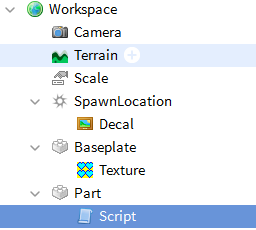# NumberValue dosent update in the script

Hello. I want a script that would set the size and position from old size, position and current NumberValue named “Scale”.

The problem is it dosent change the size and position. Further more, it dosent read the changed NumberValue!

I didnt had any working solutions yet! Can you please figure the problem out?

Workspace:Code:

``````print("starting setup")
xpos = script.Parent.Position.X
ypos = script.Parent.Position.Y
zpos = script.Parent.Position.Z
xscale = script.Parent.Size.X
yscale = script.Parent.Size.Y
zscale = script.Parent.Size.Z
value = 0
print("setup done")
while true do
value = 10^((game.Workspace.Scale.Value)-(xpos/16))
print(game.Workspace.Scale.Value)
script.Parent.Size = Vector3.new(xscale*value,yscale*value,zscale*value)
script.Parent.Position = Vector3.new(30,(script.Parent.Size.Y/2)+1,zpos*value)
wait(0)
end
``````

Output:

``````  18:25:29.435  starting setup  -  Server - Script:1
18:25:29.435  setup done  -  Server - Script:9
18:25:29.436   ▶ 0.25 (x252)  -  Server - Script:12
``````
1 Like

if `value = 10^((game.Workspace.Scale.Value)-(xpos/16))` is supposed to be stored in the NumberValue you aren’t actually setting the value of scale anywhere!
Try

``````value = 10^((game.Workspace.Scale.Value)-(xpos/16))
workspace.Scale.Value = value
print(game.Workspace.Scale.Value)
``````
1 Like